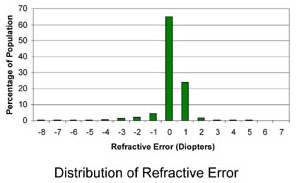## Archive for June, 2013

### tetration

Here is a problem from fakalin. Find the error in the following proof:

Let $$x^{x^{x\cdots}} = 2$$. Then $$x^{x^{x\cdots}} = x^{x^{x^{x\cdots}}} = 2$$. Substituting, we get $$x^2=2$$ and $$x=\sqrt{2}$$. Now let $$x^{x^{x\cdots}} = 4$$. Similarly, $$x^{x^{x^{x\cdots}}} = 4$$, $$x^4=4$$ and $$x=\sqrt{2}$$. But then $$2=x^{x^{x\cdots}}=4$$ and $$2=4$$.According to this distribution, the mild amount of hyperopia (far-sightedness) I have in both eyes is in statistically acceptable ranges, and is easily corrected optically. Even without correction, this amount of hyperopia — unlike myopia — is such that I could and did get away with normal accommodation. After a visit to a very thorough ophthalmologist, though, I found out that refractive error was the least interesting thing about my vision, and as a result I finally have answers to everything that I had wondered about for years. For example, now I know why it was more difficult for me to see those Magic Eye images.